# pie

## Syntax

``pie(X)``
``pie(X,explode)``
``pie(X,labels)``
``pie(X,explode,labels)``
``pie(ax,___)``
``p = pie(___)``

## Description

example

````pie(X)` draws a pie chart using the data in `X`. Each slice of the pie chart represents an element in `X`.If `sum(X) ≤ 1`, then the values in `X` directly specify the areas of the pie slices. `pie` draws only a partial pie if ```sum(X) < 1```.If `sum(X) > 1`, then `pie` normalizes the values by `X/sum(X)` to determine the area of each slice of the pie.If `X` is of data type `categorical`, the slices correspond to categories. The area of each slice is the number of elements in the category divided by the number of elements in `X`.```

example

````pie(X,explode)` offsets slices from the pie. `explode` is a vector or matrix of zeros and nonzeros that correspond to `X`. The `pie` function offsets slices for the nonzero elements only in `explode`.If `X` is of data type `categorical`, then `explode` can be a vector of zeros and nonzeros corresponding to categories, or a cell array of the names of categories to offset.```

example

````pie(X,labels)` specifies text labels for the slices. `X` must be numeric. The number of labels must equal the number of elements in `X`.```

example

````pie(X,explode,labels)` offsets slices and specifies the text labels. `X` can be numeric or categorical. For numeric `X`, the number of labels must equal the number of elements in `X`. For categorical `X`, the number of labels must equal the number of categories.```

example

````pie(ax,___)` plots into the axes specified by `ax` instead of into the current axes (`gca`). The option `ax` can precede any of the input argument combinations in the previous syntaxes.```

example

````p = pie(___)` returns a vector of patch and text graphics objects. The input can be any of the input argument combinations in the previous syntaxes.```

## Examples

collapse all

Create a pie chart of vector `X`.

```X = [1 3 0.5 2.5 2]; pie(X)```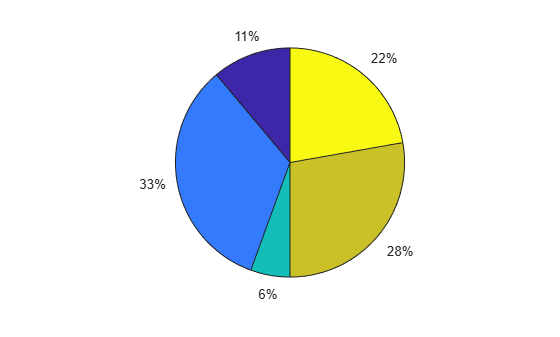Offset the second and fourth pie slices by setting the corresponding `explode` elements to 1.

```explode = [0 1 0 1 0]; pie(X,explode)```Create a pie chart of vector `X` and label the slices.

```X = 1:3; labels = {'Taxes','Expenses','Profit'}; pie(X,labels)```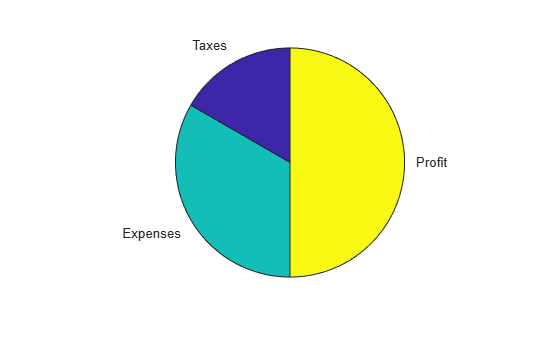Create a labeled pie chart, and then modify the color and font size of the text labels.

```X = 1:3; labels = {'Taxes','Expenses','Profit'}; p = pie(X,labels)```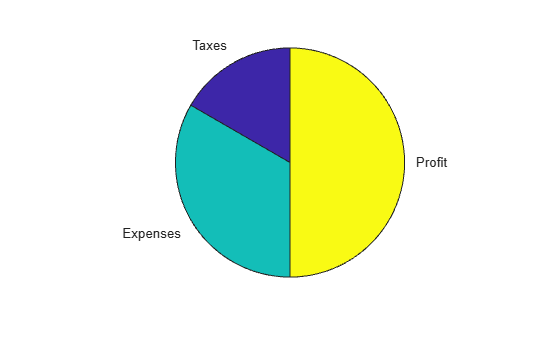```p = 1x6 graphics array: Patch Text Patch Text Patch Text ```

Get the text object for the label 'Profit'. Change its color and font size. Starting in R2014b, you can use dot notation to set properties. If you are using an earlier release, use the `set` function instead.

```t = p(6); t.BackgroundColor = 'cyan'; t.EdgeColor = 'red'; t.FontSize = 14;```Create a pie chart of vector `X` where the sum of the elements is less than 1.

```X = [0.19 0.22 0.41]; pie(X)````pie` draws a partial pie because the sum of the elements is less than 1.

Create vectors `y2010` and `y2011`, that contain financial data for two years. Then create a cell array containing the labels for the values.

```y2010 = [50 0 100 95]; y2011 = [65 22 97 120]; labels = {'Investments','Cash','Operations','Sales'};```

Create a `2`-by-`1` tiled chart layout, and display a pie chart and legend for `y2010` data in the first tile. Then display a pie chart and legend for the `y2011` data in the second tile.

```t = tiledlayout(2,1); ax1 = nexttile; pie(ax1,y2010) legend(labels) title('2010') ax2 = nexttile; pie(ax2,y2011) legend(labels) title('2011')```Plot a categorical pie chart with offset slices corresponding to categories.

```X = categorical({'North','South','North','East','South','West'}); explode = {'North','South'}; pie(X,explode)```Now, use a logical vector to offset the same slices.

```explode = [0 1 1 0]; pie(X,explode)```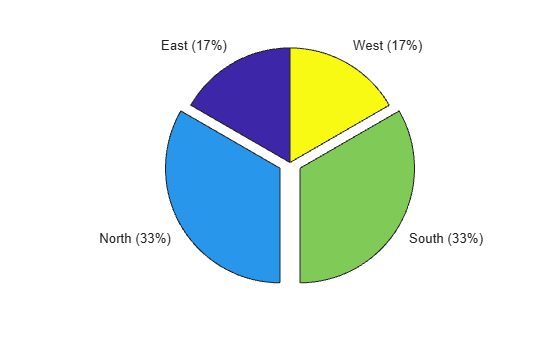Plot a categorical pie chart without any offset slices and label the slices. When `X` is of data type `categorical` you must specify the input argument `explode`. To specify labels without any offset slices, specify `explode` as an empty cell array, and `labels` as the labels.

```X = categorical({'North','South','North','East','South','West'}); explode = {}; labels = {'E','N','S','W'}; pie(X,explode,labels)```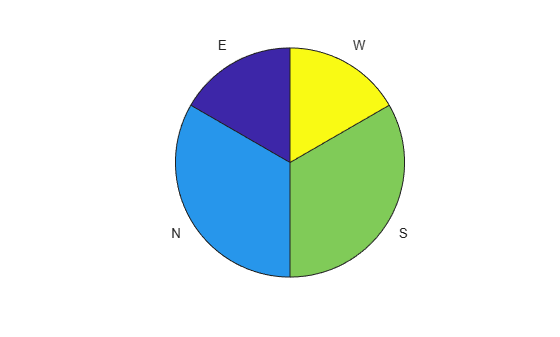Now, offset a slice and label all slices.

```X = categorical({'North','South','North','East','South','West'}); explode = {'West'}; labels = {'E','N','S','W'}; pie(X,explode,labels)```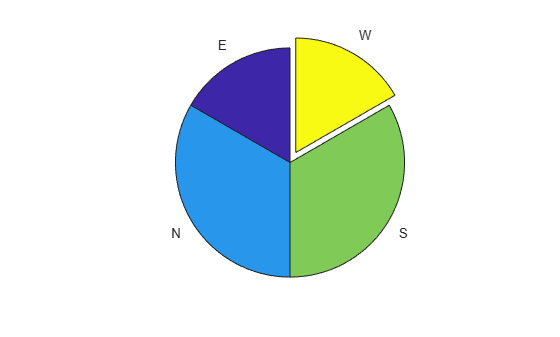## Input Arguments

collapse all

Input vector or matrix.

• If `X` is numeric, then all values in `X` must be finite.

• If `X` is categorical, then `pie` ignores undefined elements.

Data Types: `double|categorical`

Offset slices, specified as a numeric vector or matrix, a cell array of character vectors, or a string array.

• If `X` is numeric, then `explode` must be a logical or numeric vector or matrix of zeros and nonzeros that correspond to `X`. A true (nonzero) value offsets the corresponding slice from the center of the pie chart, so that `X(i,j)` is offset from the center if `explode(i,j)` is nonzero. `explode` must be the same size as `X`.

• If `X` is categorical, then `explode` can be a cell array of character vectors that are category names or a string array of category names. `pie` offsets slices corresponding to categories in `explode`.

• If `X` is categorical, then `explode` also can be a logical or numeric vector with elements that correspond to each category in `X`. The `pie` function offsets slices corresponding to true (nonzero) in category order.

Text labels for slices, specified as a cell array of character vectors or a string array.

Axes object. Use `ax` to plot the pie chart in a specific axes instead of the current axes (`gca`).

## Output Arguments

collapse all

`Patch` and `Text` objects, returned as a vector. For more information, see Patch Properties and Text Properties.

## Compatibility Considerations

expand all

Behavior changed in R2019b